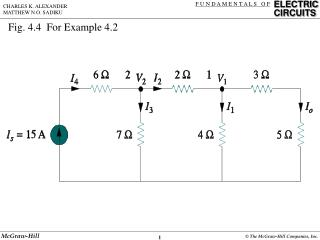DownloadDownload PresentationFig. 4.4 For Example 4.2

# Fig. 4.4 For Example 4.2

Télécharger la présentation## Fig. 4.4 For Example 4.2

- - - - - - - - - - - - - - - - - - - - - - - - - - - E N D - - - - - - - - - - - - - - - - - - - - - - - - - - -
##### Presentation Transcript

1. Fig. 4.4 For Example 4.2

2. Fig. 4.6 For Example 4.3

3. Fig. 4.7 For Example 4.3; a) calculating v1, (b) calculating v2.

4. Fig. 4.15 Transformation of independent sources.

5. Fig. 4.16 Transformation of dependent sources.

6. Fig. 4.17 for Example 4.6

7. Fig. 4.18 for Example 4.6

8. Fig. 4.23 Replacing a linear two-terminal circuit by its Thevenin equivalent: (a) original circuit, (b) the Thevenin equivalent circuit.

9. Fig. 4.23 Replacing a linear two-terminal circuit by its Thevenin equivalent: (a) original circuit, (b) the Thevenin equivalent circuit.

10. Fig. 4.24 Finding VTh and RTh.

11. Fig. 4.25 Finding RTh when circuit has dependent sources

12. Fig. 4.38 Finding Norton current IN.

13. Fig. 4.48 The circuit used for maximum power transfer.

14. Fig. 4.49 Power delivered to the load as a function of RL.